# Alon, Noga

## Arc-disjoint directed cycles in regular directed graphs ★★

Author(s): Alon; McDiarmid; Molloy

Conjecture   Ifis a-regular directed graph with no parallel arcs, thencontains a collection ofarc-disjoint directed cycles.

Keywords:

## PTAS for feedback arc set in tournaments ★★

Author(s): Ailon; Alon

Question   Is there a polynomial time approximation scheme for the feedback arc set problem for the class of tournaments?

Keywords: feedback arc set; PTAS; tournament

## List chromatic number and maximum degree of bipartite graphs ★★

Author(s): Alon

Conjecture   There is a constantsuch that the list chromatic number of any bipartite graphof maximum degreeis at most.

Keywords:

## Splitting a digraph with minimum outdegree constraints ★★★

Author(s): Alon

Problem   Is there a minimum integer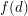such that the vertices of any digraph with minimum outdegreecan be partitioned into two classes so that the minimum outdegree of the subgraph induced by each class is at least?

Keywords:

## Nearly spanning regular subgraphs ★★★

Author(s): Alon; Mubayi

Conjecture   For everyand every positive integer, there exists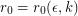so that every simple-regular graphwith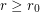has a-regular subgraphwith.

Keywords: regular; subgraph

## Even vs. odd latin squares ★★★

Author(s): Alon; Tarsi

A latin square is even if the product of the signs of all of the row and column permutations is 1 and is odd otherwise.

Conjecture   For every positive even integer, the number of even latin squares of orderand the number of odd latin squares of orderare different.

Keywords: latin square

## Ramsey properties of Cayley graphs ★★★

Author(s): Alon

Conjecture   There exists a fixed constantso that every abelian grouphas a subset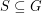withso that the Cayley graphhas no clique or independent set of size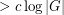.

Keywords: Cayley graph; Ramsey number

## Alon-Saks-Seymour Conjecture ★★★

Author(s): Alon; Saks; Seymour

Conjecture   Ifis a simple graph which can be written as an union ofedge-disjoint complete bipartite graphs, then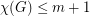.

## Bounded colorings for planar graphs ★★

Author(s): Alon; Ding; Oporowski; Vertigan

Question   Does there exists a fixed functionso that every planar graph of maximum degreehas a partition of its vertex set into at most three sets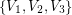so that for, every component of the graph induced byhas size at most?

Keywords: coloring; partition; planar graph

## Strong colorability ★★★

Author(s): Aharoni; Alon; Haxell

Letbe a positive integer. We say that a graphis strongly-colorable if for every partition of the vertices to sets of size at mostthere is a proper-coloring ofin which the vertices in each set of the partition have distinct colors.

Conjecture   Ifis the maximal degree of a graph, thenis strongly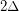-colorable.

Keywords: strong coloring